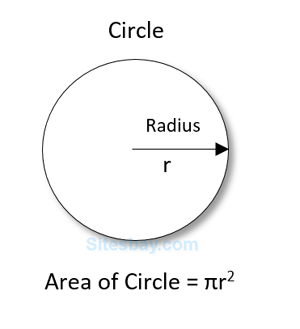# Find area of circle in C++

## Find Area of Circle Program in C++

In C++ programming you can easily write any program, for print message on screen use cout<<" " and for receive input use cin>>. For calculate area of circle you need radius of circle and apply below formula;## Formula

```area of circle = 3.14*(radius*radius);
```

## program to find area of circle

```#include<iostream.h>
#include<conio.h>

void main()
{
float area_circle=0;
clrscr();
cout<<"Arial of circle: "<<area_circle;
getch();
}
```

## Output

```Enter radius of circle: 5
Area of Circle: 78.5
```